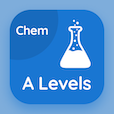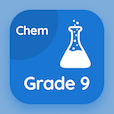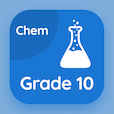Cambridge Online Courses (GCE)

A Level Chemistry MCQs

A Level Chemistry MCQ - Topics

Learn Balancing Equations Reactions with Chlorine Multiple Choice Questions (MCQ Quiz), Balancing Equations Reactions with Chlorine quiz answers PDF to learn a level chemistry course for a level chemistry online classes. Periodicity Multiple Choice Questions and Answers (MCQs), Balancing Equations Reactions with Chlorine quiz questions for online degree programs. Balancing Equations Reactions with Chlorine Book PDF: chlorides of period 3 elements, reaction of sodium and magnesium with water, oxidation number of oxides, chemical properties of chlorine test prep for GRE prep classes.

"Consider the reaction: Si(s) + Cl2(g) → SiCl4 (l). Moles of SiCl4 produced with 2 moles of Cl2 will be" MCQ PDF: balancing equations: reactions with chlorine App APK with 1, 2, 3, and 4 choices for online degree programs. Study balancing equations reactions with chlorine quiz questions for merit scholarship test and certificate programs for best online ACT prep class.

## MCQs on Balancing Equations Reactions with Chlorine Quiz

MCQ: Consider the reaction: Si(s) + Cl2(g) → SiCl4 (l). Moles of SiCl4 produced with 2 moles of Cl2 will be

1
2
3
4

MCQ: Consider the reaction: Al(s) + Cl2(g) → Al2Cl6(s). Moles of Al(s) needed to balance the equation will be

1
2
3
4

### More Quizzes on A Level Chemistry Book